@hanxiaoyang 2017-12-14T04:42:20.000000Z 字数 15073 阅读 3086

# 机器学习系列(12)_XGBoost参数调优完全指南（附Python代码）

个人博客

1、XGBoost的优势
2、理解XGBoost的参数
3、调参示例

## 4. XGBoost的优势

XGBoost算法可以给预测模型带来能力的提升。当我对它的表现有更多了解的时候，当我对它的高准确率背后的原理有更多了解的时候，我发现它具有很多优势：

### 4.1 正则化

• 标准GBM的实现没有像XGBoost这样的正则化步骤。正则化对减少过拟合也是有帮助的。
• 实际上，XGBoost以“正则化提升(regularized boosting)”技术而闻名。

### 4.2 并行处理

• XGBoost可以实现并行处理，相比GBM有了速度的飞跃。
• 不过，众所周知，Boosting算法是顺序处理的，它怎么可能并行呢？每一课树的构造都依赖于前一棵树，那具体是什么让我们能用多核处理器去构造一个树呢？我希望你理解了这句话的意思。如果你希望了解更多，点击这个链接

### 4.3 高度的灵活性

• XGBoost 允许用户定义自定义优化目标和评价标准
• 它对模型增加了一个全新的维度，所以我们的处理不会受到任何限制。

### 4.4 缺失值处理

• XGBoost内置处理缺失值的规则。
• 用户需要提供一个和其它样本不同的值，然后把它作为一个参数传进去，以此来作为缺失值的取值。XGBoost在不同节点遇到缺失值时采用不同的处理方法，并且会学习未来遇到缺失值时的处理方法。

### 4.5 剪枝

• 当分裂时遇到一个负损失时，GBM会停止分裂。因此GBM实际上是一个贪心算法
• XGBoost会一直分裂到指定的最大深度(max_depth)，然后回过头来剪枝。如果某个节点之后不再有正值，它会去除这个分裂。
• 这种做法的优点，当一个负损失（如-2）后面有个正损失（如+10）的时候，就显现出来了。GBM会在-2处停下来，因为它遇到了一个负值。但是XGBoost会继续分裂，然后发现这两个分裂综合起来会得到+8，因此会保留这两个分裂。

### 4.6 内置交叉验证

• XGBoost允许在每一轮boosting迭代中使用交叉验证。因此，可以方便地获得最优boosting迭代次数。
• 而GBM使用网格搜索，只能检测有限个值。

### 4.7、在已有的模型基础上继续

• XGBoost可以在上一轮的结果上继续训练。这个特性在某些特定的应用上是一个巨大的优势。
• sklearn中的GBM的实现也有这个功能，两种算法在这一点上是一致的。

XGBoost Guide - Introduce to Boosted Trees
Words from the Auther of XGBoost [Viedo]

## 5. XGBoost的参数

XGBoost的作者把所有的参数分成了三类：

1. 通用参数：宏观函数控制。
2. Booster参数：控制每一步的booster(tree/regression)。
3. 学习目标参数：控制训练目标的表现。

### 5.1 通用参数

#### 1、booster[默认gbtree]

• 选择每次迭代的模型，有两种选择：
gbtree：基于树的模型
gbliner：线性模型

#### 2、silent[默认0]

• 当这个参数值为1时，静默模式开启，不会输出任何信息。
• 一般这个参数就保持默认的0，因为这样能帮我们更好地理解模型。

• 这个参数用来进行多线程控制，应当输入系统的核数。
• 如果你希望使用CPU全部的核，那就不要输入这个参数，算法会自动检测它。

### 5.2 booster参数

#### 1、eta[默认0.3]

• 和GBM中的 learning rate 参数类似。
• 通过减少每一步的权重，可以提高模型的鲁棒性。
• 典型值为0.01-0.2。

#### 2、min_child_weight[默认1]

• 决定最小叶子节点样本权重和。
• 和GBM的 min_child_leaf 参数类似，但不完全一样。XGBoost的这个参数是最小样本权重的和，而GBM参数是最小样本总数
• 这个参数用于避免过拟合。当它的值较大时，可以避免模型学习到局部的特殊样本。
• 但是如果这个值过高，会导致欠拟合。这个参数需要使用CV来调整。

#### 3、max_depth[默认6]

• 和GBM中的参数相同，这个值为树的最大深度。
• 这个值也是用来避免过拟合的。max_depth越大，模型会学到更具体更局部的样本。
• 需要使用CV函数来进行调优。
• 典型值：3-10

#### 4、max_leaf_nodes

• 树上最大的节点或叶子的数量。
• 可以替代max_depth的作用。因为如果生成的是二叉树，一个深度为n的树最多生成$n^2$个叶子。
• 如果定义了这个参数，GBM会忽略max_depth参数。

#### 5、gamma[默认0]

• 在节点分裂时，只有分裂后损失函数的值下降了，才会分裂这个节点。Gamma指定了节点分裂所需的最小损失函数下降值。
• 这个参数的值越大，算法越保守。这个参数的值和损失函数息息相关，所以是需要调整的。

#### 6、max_delta_step[默认0]

• 这参数限制每棵树权重改变的最大步长。如果这个参数的值为0，那就意味着没有约束。如果它被赋予了某个正值，那么它会让这个算法更加保守。
• 通常，这个参数不需要设置。但是当各类别的样本十分不平衡时，它对逻辑回归是很有帮助的。
• 这个参数一般用不到，但是你可以挖掘出来它更多的用处。

#### 7、subsample[默认1]

• 和GBM中的subsample参数一模一样。这个参数控制对于每棵树，随机采样的比例。
• 减小这个参数的值，算法会更加保守，避免过拟合。但是，如果这个值设置得过小，它可能会导致欠拟合。
• 典型值：0.5-1

#### 8、colsample_bytree[默认1]

• 和GBM里面的max_features参数类似。用来控制每棵随机采样的列数的占比(每一列是一个特征)。
• 典型值：0.5-1

#### 9、colsample_bylevel[默认1]

• 用来控制树的每一级的每一次分裂，对列数的采样的占比。
• 我个人一般不太用这个参数，因为subsample参数和colsample_bytree参数可以起到相同的作用。但是如果感兴趣，可以挖掘这个参数更多的用处。

#### 10、lambda[默认1]

• 权重的L2正则化项。(和Ridge regression类似)。
• 这个参数是用来控制XGBoost的正则化部分的。虽然大部分数据科学家很少用到这个参数，但是这个参数在减少过拟合上还是可以挖掘出更多用处的。

#### 11、alpha[默认1]

• 权重的L1正则化项。(和Lasso regression类似)。
• 可以应用在很高维度的情况下，使得算法的速度更快。

#### 12、scale_pos_weight[默认1]

• 在各类别样本十分不平衡时，把这个参数设定为一个正值，可以使算法更快收敛。

### 5.3学习目标参数

#### 1、objective[默认reg:linear]

• 这个参数定义需要被最小化的损失函数。最常用的值有：
• binary:logistic 二分类的逻辑回归，返回预测的概率(不是类别)。
• multi:softmax 使用softmax的多分类器，返回预测的类别(不是概率)。
• 在这种情况下，你还需要多设一个参数：num_class(类别数目)。
• multi:softprob 和multi:softmax参数一样，但是返回的是每个数据属于各个类别的概率。

#### 2、eval_metric[默认值取决于objective参数的取值]

• 对于有效数据的度量方法。
• 对于回归问题，默认值是rmse，对于分类问题，默认值是error。
• 典型值有：
• rmse 均方根误差($\sqrt \frac{\sum_{i=1}^N \epsilon^2}{N}$)
• mae 平均绝对误差($\frac{\sum_{i=1}^N |\epsilon|}{N}$)
• logloss 负对数似然函数值
• error 二分类错误率(阈值为0.5)
• merror 多分类错误率
• mlogloss 多分类logloss损失函数
• auc 曲线下面积

#### 3、seed(默认0)

• 随机数的种子
• 设置它可以复现随机数据的结果，也可以用于调整参数

1、eta -> learning_rate
2、lambda -> reg_lambda
3、alpha -> reg_alpha

XGBoost Guide 的一些部分是我强烈推荐大家阅读的，通过它可以对代码和参数有一个更好的了解：

## 6. 调参示例

• City变量，因为类别太多，所以删掉了一些类别。
• DOB变量换算成年龄，并删除了一些数据。
• 增加了 EMI_Loan_Submitted_Missing 变量。如果EMI_Loan_Submitted变量的数据缺失，则这个参数的值为1。否则为0。删除了原先的EMI_Loan_Submitted变量。
• EmployerName变量，因为类别太多，所以删掉了一些类别。
• 因为Existing_EMI变量只有111个值缺失，所以缺失值补充为中位数0。
• 增加了 Interest_Rate_Missing 变量。如果Interest_Rate变量的数据缺失，则这个参数的值为1。否则为0。删除了原先的Interest_Rate变量。
• 删除了Lead_Creation_Date，从直觉上这个特征就对最终结果没什么帮助。
• Loan_Amount_Applied, Loan_Tenure_Applied 两个变量的缺项用中位数补足。
• 增加了 Loan_Amount_Submitted_Missing 变量。如果Loan_Amount_Submitted变量的数据缺失，则这个参数的值为1。否则为0。删除了原先的Loan_Amount_Submitted变量。
• 增加了 Loan_Tenure_Submitted_Missing 变量。如果 Loan_Tenure_Submitted 变量的数据缺失，则这个参数的值为1。否则为0。删除了原先的 Loan_Tenure_Submitted 变量。
• 删除了LoggedIn, Salary_Account 两个变量
• 增加了 Processing_Fee_Missing 变量。如果 Processing_Fee 变量的数据缺失，则这个参数的值为1。否则为0。删除了原先的 Processing_Fee 变量。
• Source前两位不变，其它分成不同的类别。
• 进行了离散化和独热编码(一位有效编码)。

#Import libraries:import pandas as pdimport numpy as npimport xgboost as xgbfrom xgboost.sklearn import XGBClassifierfrom sklearn import cross_validation, metrics   #Additional     scklearn functionsfrom sklearn.grid_search import GridSearchCV   #Perforing grid searchimport matplotlib.pylab as plt%matplotlib inlinefrom matplotlib.pylab import rcParamsrcParams['figure.figsize'] = 12, 4train = pd.read_csv('train_modified.csv')target = 'Disbursed'IDcol = 'ID'

• xgb - 直接引用xgboost。接下来会用到其中的“cv”函数。
• XGBClassifier - 是xgboost的sklearn包。这个包允许我们像GBM一样使用Grid Search 和并行处理。

def modelfit(alg, dtrain, predictors,useTrainCV=True, cv_folds=5, early_stopping_rounds=50):if useTrainCV:    xgb_param = alg.get_xgb_params()    xgtrain = xgb.DMatrix(dtrain[predictors].values, label=dtrain[target].values)    cvresult = xgb.cv(xgb_param, xgtrain, num_boost_round=alg.get_params()['n_estimators'], nfold=cv_folds,        metrics='auc', early_stopping_rounds=early_stopping_rounds, show_progress=False)    alg.set_params(n_estimators=cvresult.shape)#Fit the algorithm on the dataalg.fit(dtrain[predictors], dtrain['Disbursed'],eval_metric='auc')#Predict training set:dtrain_predictions = alg.predict(dtrain[predictors])dtrain_predprob = alg.predict_proba(dtrain[predictors])[:,1]#Print model report:print "\nModel Report"print "Accuracy : %.4g" % metrics.accuracy_score(dtrain['Disbursed'].values, dtrain_predictions)print "AUC Score (Train): %f" % metrics.roc_auc_score(dtrain['Disbursed'], dtrain_predprob)feat_imp = pd.Series(alg.booster().get_fscore()).sort_values(ascending=False)feat_imp.plot(kind='bar', title='Feature Importances')plt.ylabel('Feature Importance Score')

### 6.1 参数调优的一般方法

1. 选择较高的学习速率(learning rate)。一般情况下，学习速率的值为0.1。但是，对于不同的问题，理想的学习速率有时候会在0.05到0.3之间波动。选择对应于此学习速率的理想决策树数量。XGBoost有一个很有用的函数“cv”，这个函数可以在每一次迭代中使用交叉验证，并返回理想的决策树数量。

2. 对于给定的学习速率和决策树数量，进行决策树特定参数调优(max_depth, min_child_weight, gamma, subsample, colsample_bytree)。在确定一棵树的过程中，我们可以选择不同的参数，待会儿我会举例说明。

3. xgboost的正则化参数的调优。(lambda, alpha)。这些参数可以降低模型的复杂度，从而提高模型的表现。

4. 降低学习速率，确定理想参数。

#### 第一步：确定学习速率和tree_based 参数调优的估计器数目

1、max_depth = 5 :这个参数的取值最好在3-10之间。我选的起始值为5，但是你也可以选择其它的值。起始值在4-6之间都是不错的选择。

2、min_child_weight = 1:在这里选了一个比较小的值，因为这是一个极不平衡的分类问题。因此，某些叶子节点下的值会比较小。

3、gamma = 0: 起始值也可以选其它比较小的值，在0.1到0.2之间就可以。这个参数后继也是要调整的。

4、subsample, colsample_bytree = 0.8: 这个是最常见的初始值了。典型值的范围在0.5-0.9之间。

5、scale_pos_weight = 1: 这个值是因为类别十分不平衡。

#Choose all predictors except target & IDcolspredictors = [x for x in train.columns if x not in [target,IDcol]]xgb1 = XGBClassifier( learning_rate =0.1, n_estimators=1000, max_depth=5, min_child_weight=1, gamma=0, subsample=0.8, colsample_bytree=0.8, objective= 'binary:logistic', nthread=4, scale_pos_weight=1, seed=27)modelfit(xgb1, train, predictors)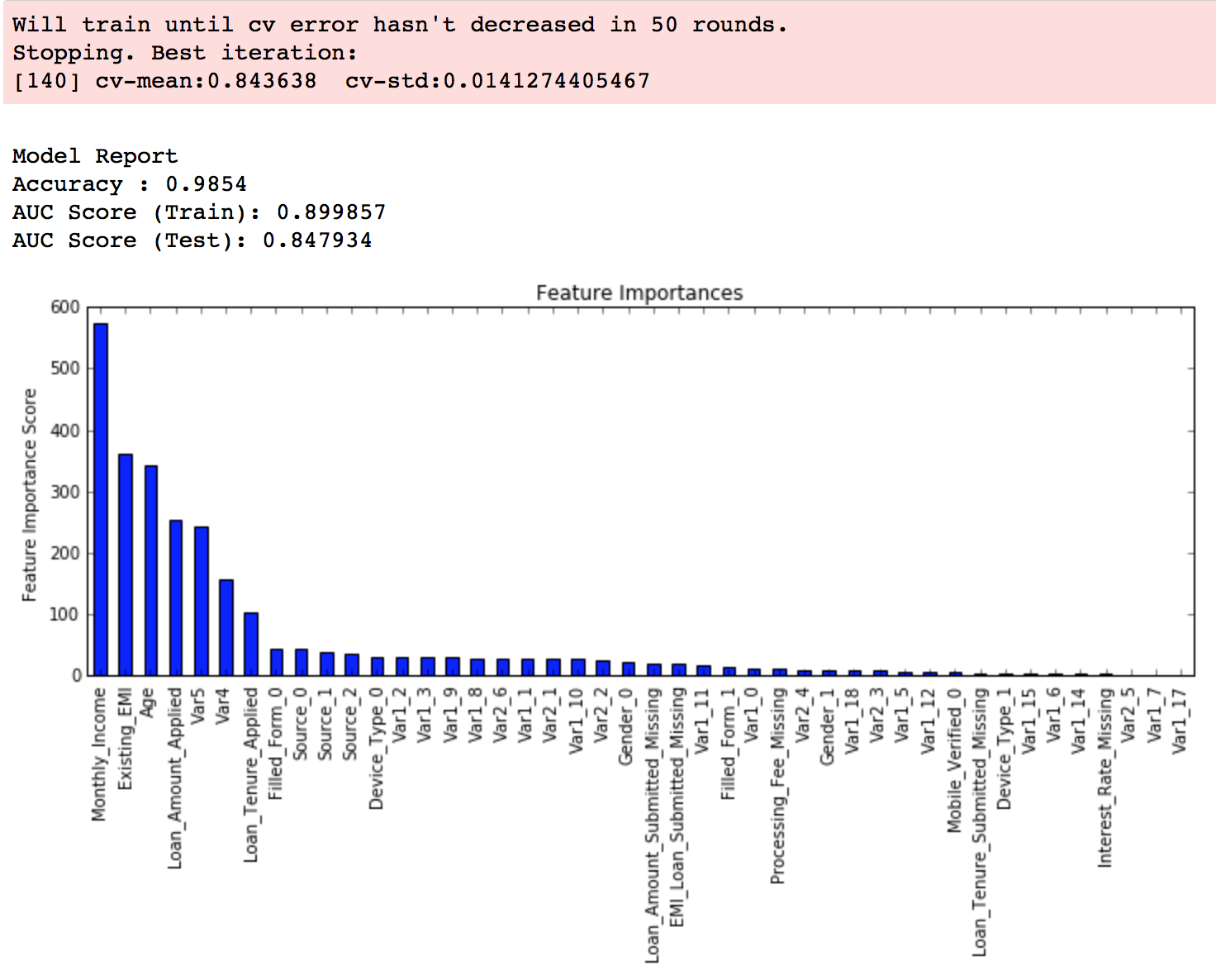#### 第二步： max_depth 和 min_weight 参数调优

param_test1 = { 'max_depth':range(3,10,2), 'min_child_weight':range(1,6,2)}gsearch1 = GridSearchCV(estimator = XGBClassifier(         learning_rate =0.1, n_estimators=140, max_depth=5,min_child_weight=1, gamma=0, subsample=0.8,             colsample_bytree=0.8, objective= 'binary:logistic', nthread=4,     scale_pos_weight=1, seed=27),  param_grid = param_test1,     scoring='roc_auc',n_jobs=4,iid=False, cv=5)gsearch1.fit(train[predictors],train[target])gsearch1.grid_scores_, gsearch1.best_params_,     gsearch1.best_score_param_test2 = { 'max_depth':[4,5,6], 'min_child_weight':[4,5,6]}gsearch2 = GridSearchCV(estimator = XGBClassifier(     learning_rate=0.1, n_estimators=140, max_depth=5, min_child_weight=2, gamma=0, subsample=0.8, colsample_bytree=0.8, objective= 'binary:logistic', nthread=4, scale_pos_weight=1,seed=27),  param_grid = param_test2, scoring='roc_auc',n_jobs=4,iid=False, cv=5)gsearch2.fit(train[predictors],train[target])gsearch2.grid_scores_, gsearch2.best_params_,     gsearch2.best_score_param_test2b = { 'min_child_weight':[6,8,10,12] }gsearch2b = GridSearchCV(estimator = XGBClassifier(     learning_rate=0.1, n_estimators=140, max_depth=4, min_child_weight=2, gamma=0, subsample=0.8, colsample_bytree=0.8, objective= 'binary:logistic', nthread=4, scale_pos_weight=1,seed=27), param_grid = param_test2b, scoring='roc_auc',n_jobs=4,iid=False, cv=5)gsearch2b.fit(train[predictors],train[target])modelfit(gsearch3.best_estimator_, train, predictors)gsearch2b.grid_scores_, gsearch2b.best_params_, gsearch2b.best_score_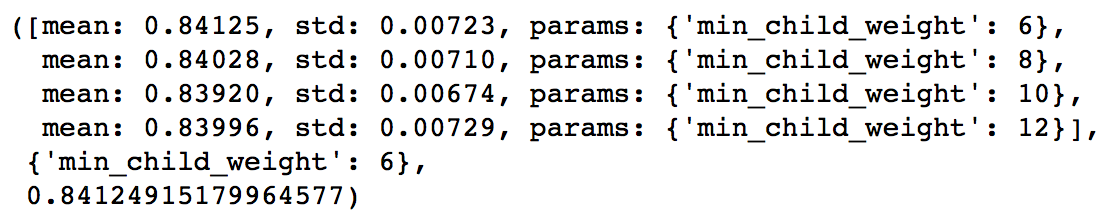#### 第三步：gamma参数调优

param_test3 = { 'gamma':[i/10.0 for i in range(0,5)]}gsearch3 = GridSearchCV(estimator = XGBClassifier( learning_rate =0.1, n_estimators=140, max_depth=4, min_child_weight=6, gamma=0, subsample=0.8, colsample_bytree=0.8, objective= 'binary:logistic', nthread=4, scale_pos_weight=1,seed=27), param_grid = param_test3, scoring='roc_auc',n_jobs=4,iid=False, cv=5)gsearch3.fit(train[predictors],train[target])gsearch3.grid_scores_, gsearch3.best_params_, gsearch3.best_score_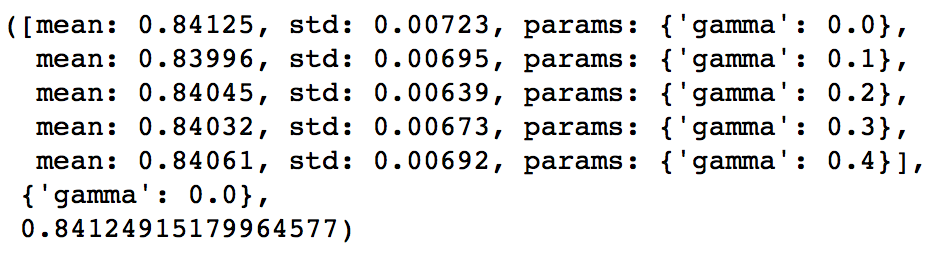xgb2 = XGBClassifier( learning_rate =0.1, n_estimators=1000, max_depth=4, min_child_weight=6, gamma=0, subsample=0.8, colsample_bytree=0.8, objective= 'binary:logistic', nthread=4,scale_pos_weight=1,seed=27)modelfit(xgb2, train, predictors)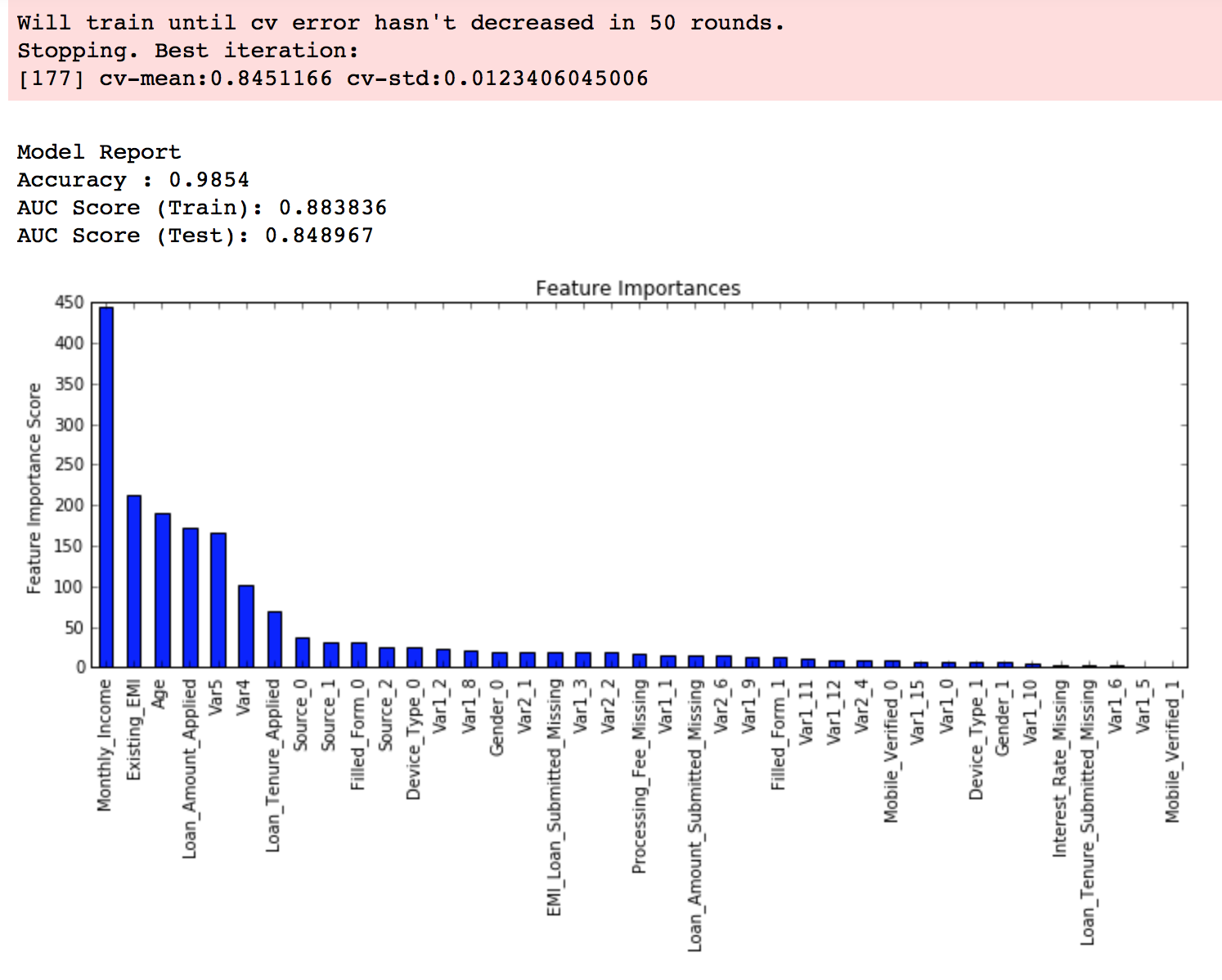# 第四步：调整subsample 和 colsample_bytree 参数

param_test4 = { 'subsample':[i/10.0 for i in range(6,10)], 'colsample_bytree':[i/10.0 for i in range(6,10)]}gsearch4 = GridSearchCV(estimator = XGBClassifier( learning_rate =0.1, n_estimators=177, max_depth=3, min_child_weight=4, gamma=0.1, subsample=0.8, colsample_bytree=0.8, objective= 'binary:logistic', nthread=4, scale_pos_weight=1,seed=27), param_grid = param_test4, scoring='roc_auc',n_jobs=4,iid=False, cv=5)gsearch4.fit(train[predictors],train[target])gsearch4.grid_scores_, gsearch4.best_params_, gsearch4.best_score_param_test5 = { 'subsample':[i/100.0 for i in range(75,90,5)], 'colsample_bytree':[i/100.0 for i in range(75,90,5)]}gsearch5 = GridSearchCV(estimator = XGBClassifier( learning_rate =0.1, n_estimators=177, max_depth=4, min_child_weight=6, gamma=0, subsample=0.8, colsample_bytree=0.8, objective= 'binary:logistic', nthread=4, scale_pos_weight=1,seed=27), param_grid = param_test5, scoring='roc_auc',n_jobs=4,iid=False, cv=5)gsearch5.fit(train[predictors],train[target])• subsample: 0.8
• colsample_bytree: 0.8

#### 第五步：正则化参数调优

param_test6 = { 'reg_alpha':[1e-5, 1e-2, 0.1, 1, 100]}gsearch6 = GridSearchCV(estimator = XGBClassifier( learning_rate =0.1, n_estimators=177, max_depth=4, min_child_weight=6, gamma=0.1, subsample=0.8, colsample_bytree=0.8, objective= 'binary:logistic', nthread=4, scale_pos_weight=1,seed=27), param_grid = param_test6, scoring='roc_auc',n_jobs=4,iid=False, cv=5)gsearch6.fit(train[predictors],train[target])gsearch6.grid_scores_, gsearch6.best_params_, gsearch6.best_score_param_test7 = { 'reg_alpha':[0, 0.001, 0.005, 0.01, 0.05]}gsearch7 = GridSearchCV(estimator = XGBClassifier( learning_rate =0.1, n_estimators=177, max_depth=4, min_child_weight=6, gamma=0.1, subsample=0.8, colsample_bytree=0.8, objective= 'binary:logistic', nthread=4, scale_pos_weight=1,seed=27), param_grid = param_test7, scoring='roc_auc',n_jobs=4,iid=False, cv=5)gsearch7.fit(train[predictors],train[target])gsearch7.grid_scores_, gsearch7.best_params_, gsearch7.best_score_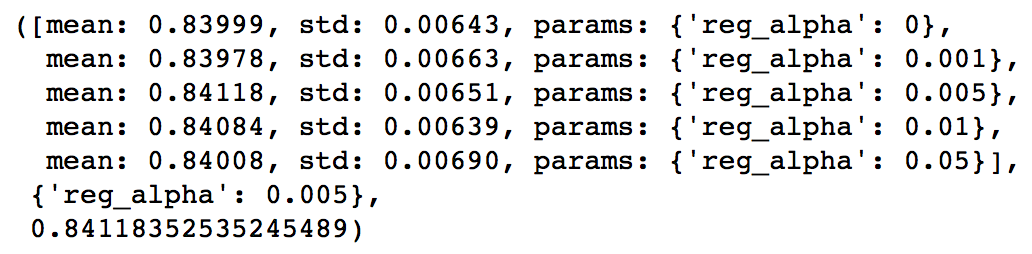xgb3 = XGBClassifier( learning_rate =0.1, n_estimators=1000, max_depth=4, min_child_weight=6, gamma=0, subsample=0.8, colsample_bytree=0.8, reg_alpha=0.005, objective= 'binary:logistic', nthread=4, scale_pos_weight=1, seed=27)modelfit(xgb3, train, predictors)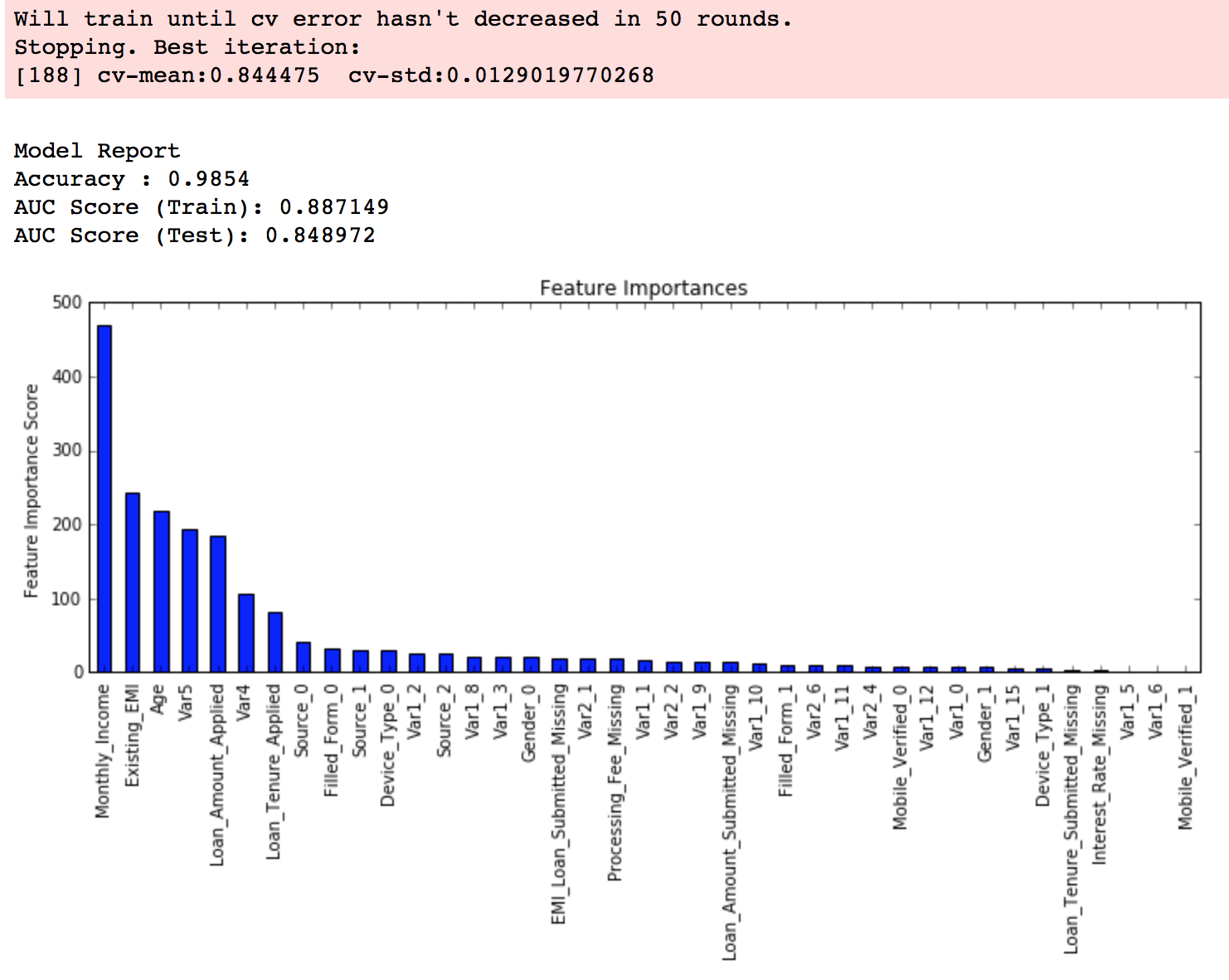#### 第6步：降低学习速率

xgb4 = XGBClassifier( learning_rate =0.01, n_estimators=5000, max_depth=4, min_child_weight=6, gamma=0, subsample=0.8, colsample_bytree=0.8, reg_alpha=0.005, objective= 'binary:logistic', nthread=4, scale_pos_weight=1, seed=27)modelfit(xgb4, train, predictors)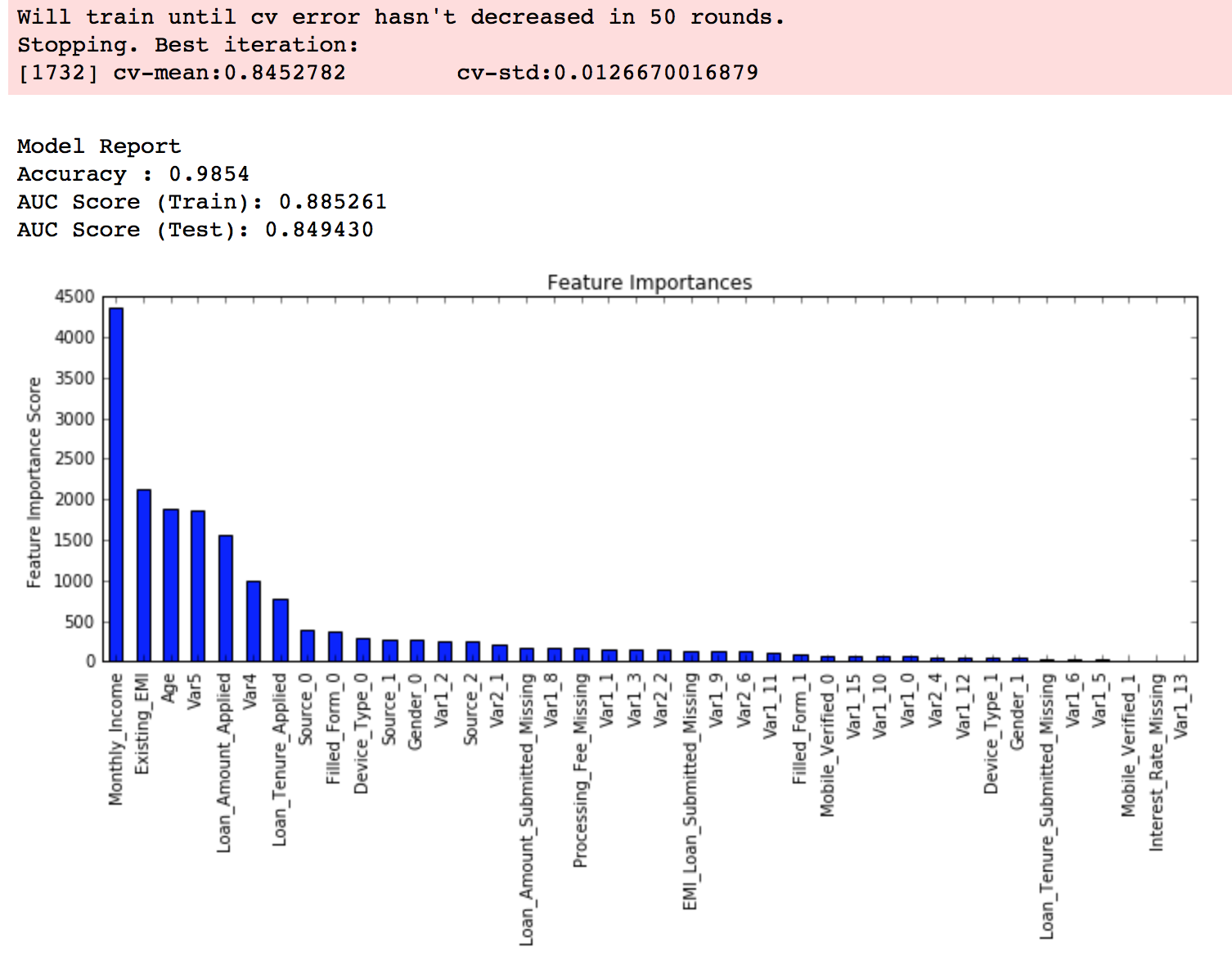1、仅仅靠参数的调整和模型的小幅优化，想要让模型的表现有个大幅度提升是不可能的。GBM的最高得分是0.8487，XGBoost的最高得分是0.8494。确实是有一定的提升，但是没有达到质的飞跃。
2、要想让模型的表现有一个质的飞跃，需要依靠其他的手段，诸如，特征工程(feature egineering) ，模型组合(ensemble of model),以及堆叠(stacking)等。

# 结束语• 私有
• 公开
• 删除